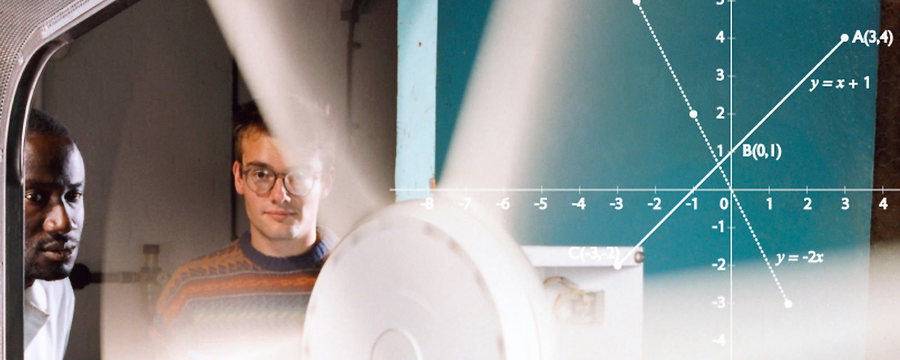•# Glossary of mathematical symbolism

This is a glossary of mathematical symbolism.© Tony Croft, Loughborough University 2014

You will find here a glossary of mathematical symbolism.

### Absolute temperature

Absolute temperature is a quantity which is important in the study of thermodynamics. It measures temperature relative to the theoretically lowest possible temperature – known as absolute zero. The SI unit of absolute temperature is the Kelvin, K. Absolute zero is defined to be (0) K, that is (-273.15^circ)C.

### Acceleration

Acceleration is the rate at which the velocity of an object is changing. If the velocity of an object is changing, then it is accelerating. Acceleration is usually measured in units of metres per second per second, or metres per second squared, written m s(^{−2}).

### Axes

The plural of axis.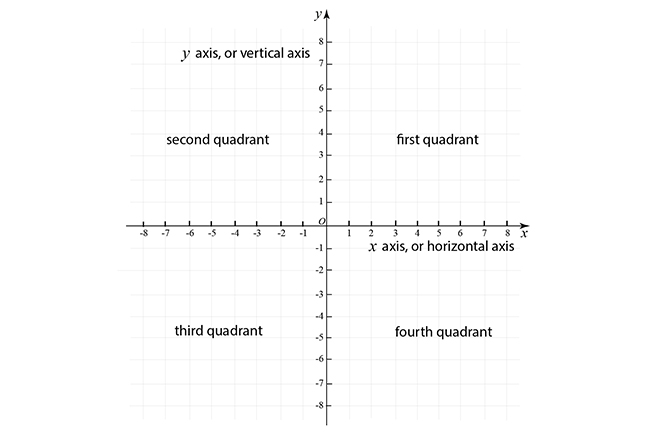### Axis

The straight line running from the left of the page to the right (called the horizontal axis, or (x) axis) or the bottom of the page to the top (called the vertical axis, or (y) axis). These two axes divide the page into four quadrants.

### Cartesian co-ordinates

A pair of numbers, written in the form ((a, b)), which specify the distance of a point from the axes. The magnitude or size of the number (a) gives the distance from the vertical axis. The magnitude or size of the number (b) gives the distance from the horizontal axis. If (a) is negative then the point is to the left of the vertical axis. If (b) is negative the point is below the horizontal axis.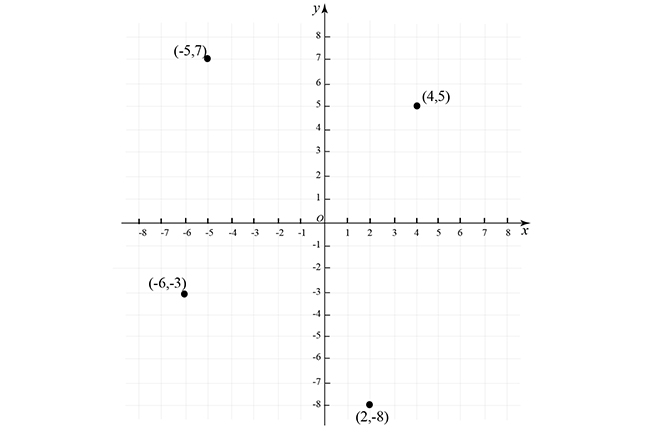### Coefficient of (x)

In equations such as (y = 3x) or (y = 8x + 7), the number multiplying the variable (x) is called the coefficient of (x). In the equation (y = mx + c) the coefficient of (x) is (m).

### Constant

A constant is a number which remains unchanged throughout the course of a mathematical problem. If the value of a constant is specified at the start, then the constant will always have that value within the context of the problem being solved.

In the equation (y = mx + c), the values of (m) and (c) are constant. The term (c) is often referred to as the constant term in the equation.

### Co-ordinates

In this course, a pair of numbers, written in the form ((a,b)), which specify the distance of a point from the axes. The magnitude or size of the number (a) gives the distance from the vertical axis. The magnitude or size of the number (b) gives the distance from the horizontal axis. If (a) is negative then the point is to the left of the vertical axis. If (b) is negative the point is below the horizontal axis. In more advanced work, other coordinate systems are possible (see Cartesian co-ordinates, above).

### Current in a circuit

In electrical engineering, the current in a circuit refers to the rate at which charge is flowing through the circuit.

### Curve

A curve is an object rather like a line, but which is not necessarily straight. (See Straight Line.)

### Dependent variable

In an equation of a straight line (y = mx + c), the variable (y) is referred to as the dependent variable because its value depends upon the value which has been chosen for (x).

### Differential calculus

Differential calculus is that branch of mathematics concerned with the rate at which a quantity changes. In physical situations variables change and it is often important to know how quickly change is occurring.

### Discontinuity

A discontinuity is a jump or break in a line or curve. Loosely speaking, when drawing a line or curve that has a discontinuity you would have to lift your pen from the paper and return it in a different position to draw the whole line or curve. A line or curve with a discontinuity is said to be discontinuous.

### Distance travelled

This is a measure of how much ground has been covered by an object during its motion.

### Equation of a line

In this course, the equation of a line is given by (y = mx + c). This is a mathematical expression which contains information about the slope of the line and where it cuts the vertical axis. This information is sufficient to specify the line completely within a given plane.

A measure of the slope of a line. Lines with positive gradient rise as we look from the left to the right. Lines with negative gradient fall. In the straight line equation (y = mx + c), the gradient of the line is (m). The larger the value of (m) the steeper is the line. Lines with zero gradient are horizontal (flat).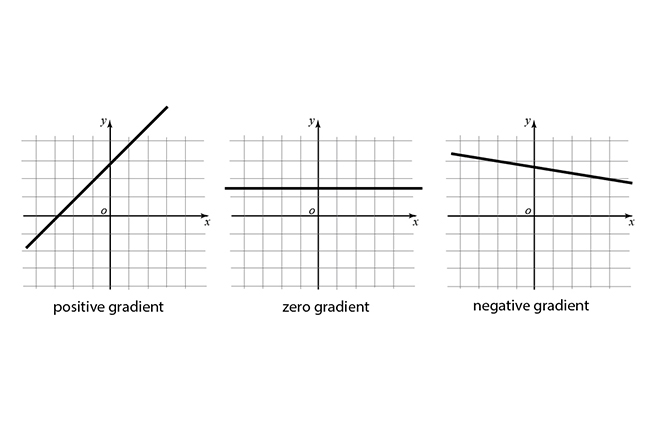### Graph

The graph of a line is a pictorial representation of the line. Usually it will comprise the horizontal and vertical axes appropriately labelled and a drawing of the line itself.

### Hooke’s Law

An experimental law attributed to the English scientist Robert Hooke (1635-1703) who discovered that under certain conditions the extension in a stretched spring or piece of elastic is proportional to the weight or load applied to cause the stretching. Equivalently, the tension force in the spring, (T), is proportional to the extension, (e), and so Hooke’s law can be written (T = ke) where (k) is a constant called the spring constant.

### Horizontal axis

A straight line running from the left of the page to the right (often called the (x) axis).

### Independent variable

In an equation of a straight line (y = mx + c), the variable (x) is referred to as the independent variable. Usually we have some choice regarding the values that the independent variable can take. However once a value for (x) is chosen, this determines the value of (y) and hence (y) is referred to as the dependent variable.

### Initial speed

The speed that an object has when we start considering its motion (at time = (0)). The initial speed is not necessarily zero. In any problem, the initial speed is a constant because even though the speed of the object may subsequently change, its initial speed does not.

### Intercept

The location where a line crosses an axis. Specifically, the vertical intercept is the location where a line crosses the vertical axis.

### Line

In this course a line is a straight line. Two distinct (or different) points in the plane of the paper are sufficient to specify a straight line which passes through them.

### Linearisation

Linearisation refers to the process of approximating a non-linear relationship – one for which the graph would be a curve for example – by a linear one. Linearisation can simplify analysis and is the basis of differential calculus.

### Linearity

Linearity refers to a relationship between variables which can be represented by a straight line. Whilst this might sound simple, the concept of linearity is fundamental to the study of vast areas of mathematics.

### Logarithm

At this level we can think of a logarithm in terms of reversing the process of ‘raising to a power’. For example, given we know that (10^2 = 10times 10 = 100), logarithms enable us to write that the logarithm (to the base 10) of 100 equals 2, written (log_{10}{100}=2). Similarly, with (2^3=8) then (log_2 8 =3). The clever thing about logarithms is that numbers which were powers no longer appear as powers. In turn this means that a non-linear relationship can be converted into a linear one.

### Mole

The mole is a unit of measurement used by chemists to express an amount of a chemical substance. The mole is one of the SI base units.

### Ohm’s law

An experimental result attributed to the German physicist Georg Simon Ohm (1789 – 1854) who discovered that under certain conditions the voltage across an electrical component called a resistor is proportional to the current flowing through it.

### Origin

The location in the plane where the horizontal and vertical axes cross. The origin has co-ordinates ((0,0)).

### Periodicity

Periodicity refers to a characteristic of a graph wherein a pattern repeats regularly. A graph consisting of a repeating pattern is said to be periodic.

### Perpendicular

Two straight lines are perpendicular if they intersect at right angles – that is at (90^circ).

### Plane

In this course you can think of a plane as the piece of paper upon which you are writing and drawing graphs. A plane has two dimensions. Another way of thinking about a plane is that it is a flat surface, and any two points on the surface can be joined with a straight line which lies entirely on the plane.

### Point

A point is a specific location in the plane.

In a two-dimensional Cartesian co-ordinate system a quadrant is one of the four parts into which the plane is divided by the axes (see Cartesian co-ordinates, above). Every point in the plane lies on an axis or in one of the four quadrants.

### Reciprocal

To find the reciprocal of a number, you divide 1 by that number. For example, the reciprocal of 2 is (frac{1}{2}) and the reciprocal of (8) is (frac{1}{8}). Observe that if a number is multiplied by its reciprocal the result is 1, as in (8 times frac{1}{8} =1). The reciprocal of a variable (x) is (frac{1}{x}).

### Regression

Regression is a statistical technique for exploring the relationship between variables. Linear regression is concerned with exploring the extent to which a relationship is linear.

### Scale

A way of labelling an axis so that distances along the axis can be measured. Scales can be chosen in various ways to suit the purpose. For example a scale may consist of whole numbers, . . . , (−3, −2, −1, 0, 1, 2, 3) . . .. It may consist of multiples, for example (0, 10, 20, 30, 40,) . . .. In this course all scales are uniform – this means that the distance between tick marks or labels is always the same.

### Scatter diagram

A scatter diagram or scatter graph is a way of graphically representing data using Cartesian co-ordinates. Drawing a scatter diagram is usually the first stage in carrying out a regression analysis to explore the relationship between a pair of variables.

### Segment

See Straight line segment.

### Slope of a line

A measure of the steepness of a line. Also called the gradient of the line. Lines with positive slope rise as we look from the left to the right. Lines with negative slope fall. In the straight line equation (y = mx + c), the slope of the line is (m). The larger the value of (m) the steeper is the line. Lines with zero slope are horizontal (flat) (see gradient of a line, above).

### Speed

In everyday life, speed is the distance travelled by an object per unit of time. Mathematically, speed is the magnitude of the velocity. When specifying the velocity of an object, in addition to speed, we should also specify the direction of travel. Thus velocity is referred to as a vector quantity whereas speed is a scalar quantity.

### Straight line

If we take a plane or flat piece of paper then any two distinct points in the plane, P and Q say, can be joined by many different curves. That curve with the shortest length is a straight line. Loosely speaking, a straight line won’t have any wiggles. A straight line can be continued indefinitely beyond its two points. We refer to part of the straight line as a straight line segment. A unique straight line can be drawn through two distinct points. An infinite number of straight lines can be drawn through a single point.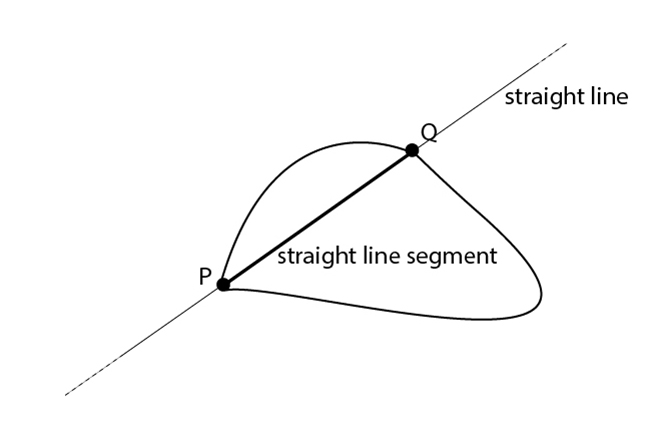### Straight line segment

A straight line segment is part of a straight line. In some applications, particularly in electrical engineering, a graph is built from straight line segments.

### Strain

Strain is a technical term related to the extension which is caused when a material is stressed, for example compressed or stretched. It is often given the symbol (epsilon). Strain is related to the stress, (sigma), which causes that strain. In some circumstances this relationship is linear and takes the form (epsilon = frac{1}{E}sigma), or equivalently, (sigma = Eepsilon) where (E) is a constant (for the particular material) called the Young’s modulus.

### Stress

Stress is a technical term related to the forces which are present in a material which is strained – compressed or stretched. (Technically, stress is defined to be the force per unit area.) It is often given the symbol (sigma). Stress is related to the strain, (epsilon). In some circumstances this relationship is linear and takes the form (sigma = Eepsilon) where (E) is a constant (for the particular material) called the Young’s modulus.

### Subscript

A subscript is a small character – number(s) or other symbol(s) – written to the bottom right of a variable in order to distinguish different instances of that variable. For instance, if the average temperature, (T), were recorded on each day of the week we might choose to label these temperatures

(T_m), (T_t), (T_w), (T_{th}), (T_f)

where (T_m) is the temperature on Monday, (T_t) is the temperature on Tuesday and so on. Similarly, if we want to record a journey time on four different occasions we might choose to refer to these as

(t_1, t_2, t_3, t_4).

Here, (m),(t),(w),(th),(f), and (1),(2),(3),(4) are subscripts.

### Substitute

In a mathematical expression involving symbols, to substitute means to replace the symbol by a specific numerical value. For example in the expression (4x + 2), substituting (x) with the specific value (3) we obtain (4 × 3 + 2 = 12 + 2 = 14).

### Superscript

A superscript is a small character – number(s) or other symbol(s) – written slightly higher and to the right of a variable. Superscripts are used to distinguish different instances of that variable in the same way that subscripts are used. So, for example, we might refer to four temperatures as

(T^0,T^1,T^2,T^3).

Such usage can be confusing because superscripts are also used to indicate powers of numbers, for example (2^3 = 2 × 2 × 2 = 8). Further, superscripts are also used to indicate angles in degrees, as in (90^◦) and temperatures, as in (212^◦)F or (100^◦)C. Often the precise meaning needs to be inferred from the context.

### Symbol

A symbol is something which stands for, or represents, something else. In this course symbols are often letters of the English or Greek alphabets used to represent physical quantities such as speed. Another type of symbol is a graph. Graphs are often used to represent relationships between quantities.

### Tension

Tension is a force, for example the force in a rope when it is used to pull an object. It is also the force in a spring which is caused by stretching the spring.

### Variable

A mathematical quantity which is allowed to vary or change. This distinguishes the quantity from a constant which is fixed and does not change.

### Velocity

Velocity is the rate at which an object’s position changes. Specifying a velocity is equivalent to specifying the object’s speed and its direction of travel.

### Vertical axis

A straight line running from the bottom of the page to the top (often called the (y) axis).

### Vertical intercept

The point or location where a line cross the vertical axis. In the equation (y = mx + c) the vertical intercept is given by the value of (c).

### Voltage across a resistor

A quantity in electrical engineering which measures the electrical tension or pressure across a component called a resistor. It is often referred to as electric potential. Negatively charged particles are attracted towards higher voltages.

### (x) axis

A straight line running from the left of the page to the right (often called the horizontal axis).

### (x) co-ordinate

Given a pair of co-ordinates ((a,b)), the first number, (a), is the (x) co-ordinate.

### (y) axis

A straight line running from the bottom of the page to the top (often called the vertical axis).

### (y) co-ordinate

Given a pair of co-ordinates ((a,b)), the second number, (b), is the (y) co-ordinate.

### Young’s modulus

Young’s modulus, (E), is a quantity which measures the stiffness of a material. It appears in the relationship between stress, (sigma), and strain, (epsilon), – often a linear relationship of the form (sigma = Eepsilon).

© Tony Croft, Loughborough University 2014

#### Getting a Grip on Mathematical Symbolism